## Minimization Of Boolean Expressions-

There are following two methods of minimizing or reducing the boolean expressions-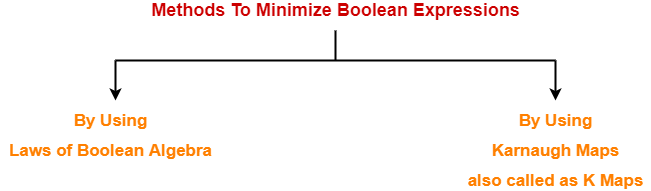1. By using laws of Boolean Algebra
2. By using Karnaugh Maps also called as K Maps

## Karnaugh Map-

 The Karnaugh Map also called as K Map is a graphical representationthat provides a systematic method for simplifying the boolean expressions.

For a boolean expression consisting of n-variables, number of cells required in K Map = 2n cells.

### Two Variable K Map-

• Two variable K Map is drawn for a boolean expression consisting of two variables.
• The number of cells present in two variable K Map = 22 = 4 cells.
• So, for a boolean function consisting of two variables, we draw a 2 x 2 K Map.

Two variable K Map may be represented as-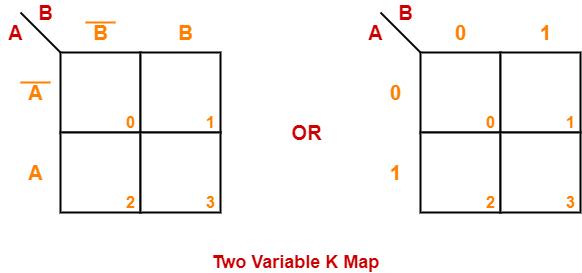Here, A and B are the two variables of the given boolean function.

### Three Variable K Map-

• Three variable K Map is drawn for a boolean expression consisting of three variables.
• The number of cells present in three variable K Map = 23 = 8 cells.
• So, for a boolean function consisting of three variables, we draw a 2 x 4 K Map.

Three variable K Map may be represented as-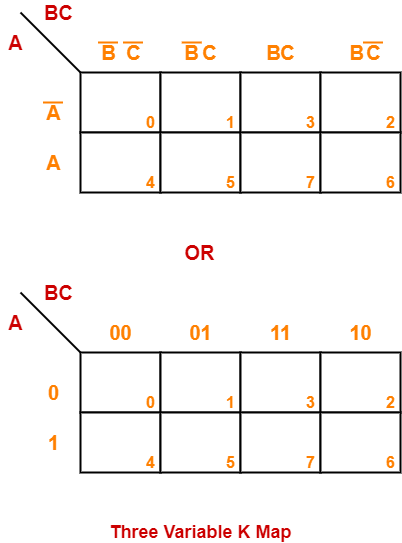Here, A, B and C are the three variables of the given boolean function.

### Four Variable K Map-

• Four variable K Map is drawn for a boolean expression consisting of four variables.
• The number of cells present in four variable K Map = 24 = 16 cells.
• So, for a boolean function consisting of four variables, we draw a 4 x 4 K Map.

Four variable K Map may be represented as-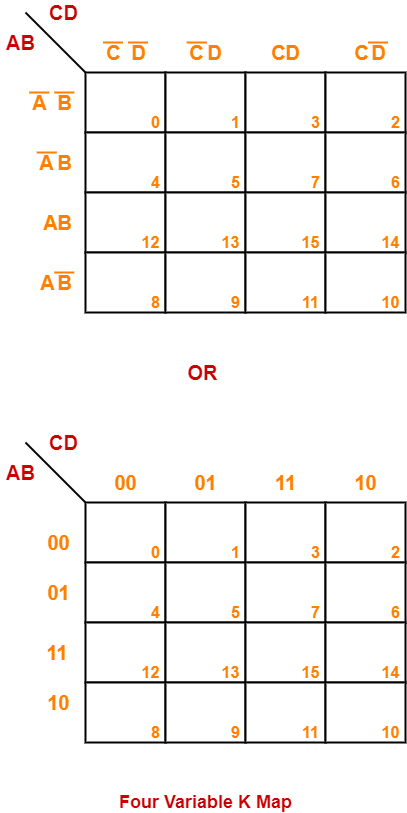Here, A, B, C and D are the four variables of the given boolean function.

## Karnaugh Map Simplification Rules-

To minimize the given boolean function,

• We draw a K Map according to the number of variables it contains.
• We fill the K Map with 0’s and 1’s according to its function.
• Then, we minimize the function in accordance with the following rules.

### Rule-01:

• We can either group 0’s with 0’s or 1’s with 1’s but we can not group 0’s and 1’s together.
• X representing don’t care can be grouped with 0’s as well as 1’s.

### NOTE

There is no need of separately grouping X’s i.e. they can be ignored if all 0’s and 1’s are already grouped.

### Rule-02:

• Groups may overlap each other.

### Rule-03:

• We can only create a group whose number of cells can be represented in the power of 2.
• In other words, a group can only contain 2n i.e. 1, 2, 4, 8, 16 and so on number of cells.

### Example-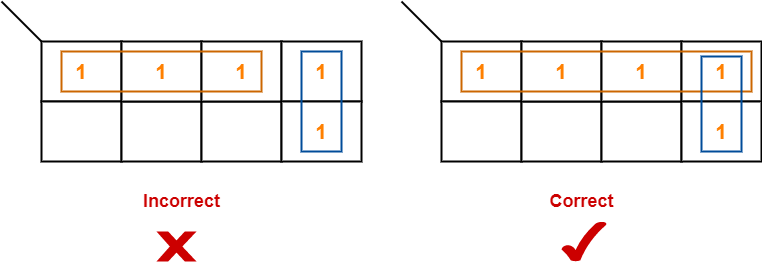### Rule-04:

• Groups can be only either horizontal or vertical.
• We can not create groups of diagonal or any other shape.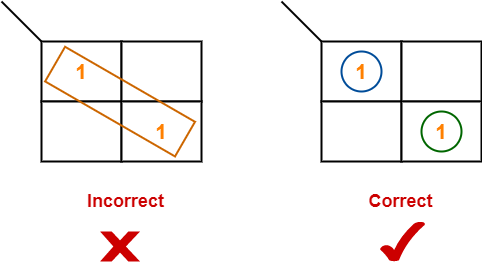### Rule-05:

• Each group should be as large as possible.

### Example-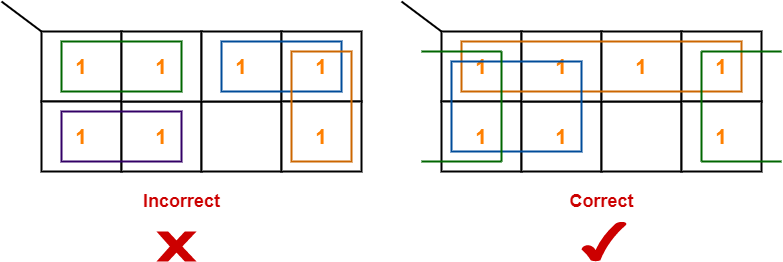### Rule-06:

• Opposite grouping and corner grouping are allowed.
• The example of opposite grouping is shown illustrated in Rule-05.
• The example of corner grouping is shown below.

### Example-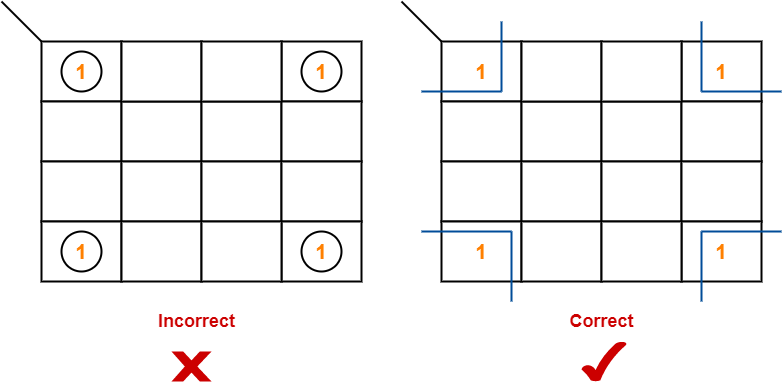### Rule-07:

• There should be as few groups as possible.

## Problem-01:

Minimize the following boolean function-

F(A, B, C, D) = Σm(0, 1, 2, 5, 7, 8, 9, 10, 13, 15)

## Solution-

• Since the given boolean expression has 4 variables, so we draw a 4 x 4 K Map.
• We fill the cells of K Map in accordance with the given boolean function.
• Then, we form the groups in accordance with the above rules.

Then, we have-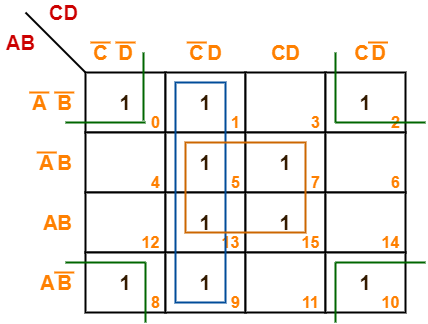Now,

F(A, B, C, D)

= (A’B + AB)(C’D + CD) + (A’B’ + A’B + AB + AB’)C’D + (A’B’ + AB’)(C’D’ + CD’)

= BD + C’D + B’D’

Thus, minimized boolean expression is-

F(A, B, C, D) = BD + C’D + B’D’

## Problem-02:

Minimize the following boolean function-

F(A, B, C, D) = Σm(0, 1, 3, 5, 7, 8, 9, 11, 13, 15)

## Solution-

• Since the given boolean expression has 4 variables, so we draw a 4 x 4 K Map.
• We fill the cells of K Map in accordance with the given boolean function.
• Then, we form the groups in accordance with the above rules.

Then, we have-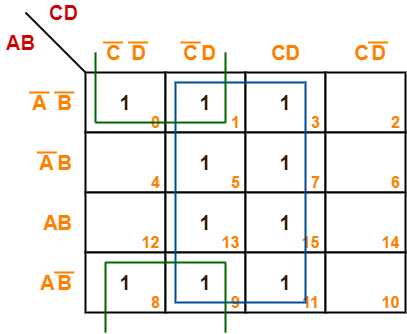Now,

F(A, B, C, D)

= (A’B’ + A’B + AB + AB’)(C’D + CD) + (A’B’ + AB’)(C’D’ + C’D)

= D + B’C’

Thus, minimized boolean expression is-

F(A, B, C, D) = B’C’ + D

## Problem-03:

Minimize the following boolean function-

F(A, B, C, D) = Σm(1, 3, 4, 6, 8, 9, 11, 13, 15) + Σd(0, 2, 14)

## Solution-

• Since the given boolean expression has 4 variables, so we draw a 4 x 4 K Map.
• We fill the cells of K Map in accordance with the given boolean function.
• Then, we form the groups in accordance with the above rules.

Then, we have-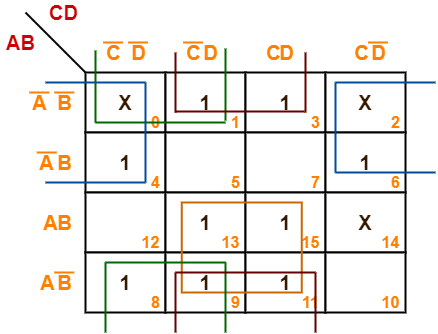Now,

F(A, B, C, D)

= (AB + AB’)(C’D + CD) + (A’B’ + AB’)(C’D + CD) + (A’B’ + AB’)(C’D’ + C’D) + (A’B’ + A’B)(C’D’ + CD’)

= AD + B’D + B’C’ + A’D’

Thus, minimized boolean expression is-

F(A, B, C, D) = AD + B’D + B’C’ + A’D’

## Problem-04:

Minimize the following boolean function-

F(A, B, C) = Σm(0, 1, 6, 7) + Σd(3, 5)

## Solution-

• Since the given boolean expression has 3 variables, so we draw a 2 x 4 K Map.
• We fill the cells of K Map in accordance with the given boolean function.
• Then, we form the groups in accordance with the above rules.

Then, we have-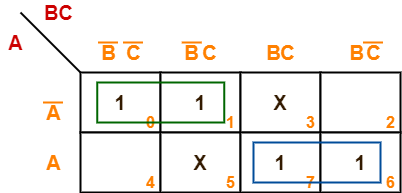Now,

F(A, B, C)

= A'(B’C’ + B’C) + A(BC + BC’)

= A’B’ + AB

Thus, minimized boolean expression is-

F(A, B, C) = AB + A’B’

### NOTE-

• It may be noted that there is no need of considering the quad group.
• This is because even if we consider that group, we will have to consider the other two duets.
• So, there is no use of considering that quad group.

## Problem-05:

Minimize the following boolean function-

F(A, B, C) = Σm(1, 2, 5, 7) + Σd(0, 4, 6)

## Solution-

• Since the given boolean expression has 3 variables, so we draw a 2 x 4 K Map.
• We fill the cells of K Map in accordance with the given boolean function.
• Then, we form the groups in accordance with the above rules.

Then, we have-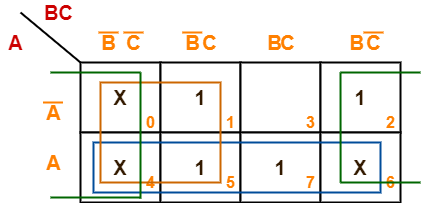Now,

F(A, B, C)

= (A + A’)(B’C’ + B’C) + A(B’C’ + B’C + BC + BC’) + (A + A’)(B’C’ + BC’)

= B’ + A + C’

Thus, minimized boolean expression is-

F(A, B, C) = A + B’ + C’

## Problem-06:

Minimize the following boolean function-

F(A, B, C) = Σm(0, 1, 6, 7) + Σd(3, 4, 5)

## Solution-

• Since the given boolean expression has 3 variables, so we draw a 2 x 4 K Map.
• We fill the cells of K Map in accordance with the given boolean function.
• Then, we form the groups in accordance with the above rules.

Then, we have-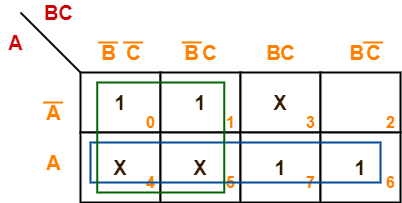Now,

F(A, B, C)

= (A + A’)(B’C’ + B’C) + A(B’C’ + B’C + BC + BC’)

= B’ + A

Thus, minimized boolean expression is-

F(A, B, C) = A + B’

## Problem-07:

Minimize the following boolean function-

F(A, B, C, D) = Σm(0, 2, 8, 10, 14) + Σd(5, 15)

## Solution-

• Since the given boolean expression has 4 variables, so we draw a 4 x 4 K Map.
• We fill the cells of K Map in accordance with the given boolean function.
• Then, we form the groups in accordance with the above rules.

Then, we have-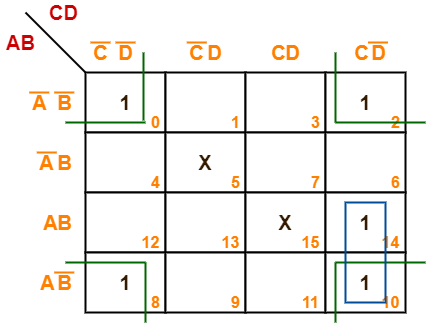Now,

F(A, B, C, D)

= (AB + AB’)CD’ + (A’B’ + AB’)(C’D’ + CD’)

= ACD’ + B’D’

Thus, minimized boolean expression is-

F(A, B, C, D) = ACD’ + B’D’

## Problem-08:

Minimize the following boolean function-

F(A, B, C, D) = Σm(3, 4, 5, 7, 9, 13, 14, 15)

## Solution-

• Since the given boolean expression has 4 variables, so we draw a 4 x 4 K Map.
• We fill the cells of K Map in accordance with the given boolean function.
• Then, we form the groups in accordance with the above rules.

Then, we have-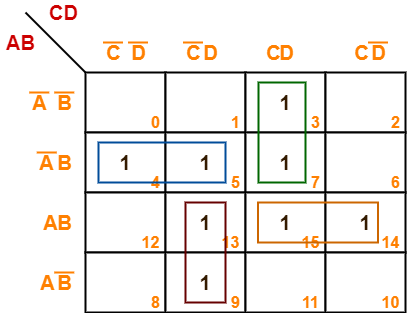Now,

F(A, B, C, D)

= A’B(C’D’ + C’D) + (A’B’ + A’B)(CD) + (AB + AB’)(C’D) + AB(CD + CD’)

= A’BC’ + A’CD + AC’D + ABC

Thus, minimized boolean expression is-

F(A, B, C, D) = A’BC’ + A’CD + AC’D + ABC

It is important to note that we are not considering the quad group because we have to consider the duets anyhow.

## Problem-09:

Consider the following boolean function-

F(W, X, Y, Z) = Σm(1, 3, 4, 6, 9, 11, 12, 14)

This function is independent ________ number of variables. Fill in the blank.

## Solution-

• Since the given boolean expression has 4 variables, so we draw a 4 x 4 K Map.
• We fill the cells of K Map in accordance with the given boolean function.
• Then, we form the groups in accordance with the above rules.

Then, we have-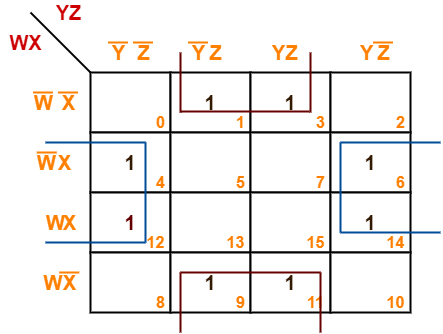Now,

F(W, X, Y, Z)

= (W’X + WX)(Y’Z’ + YZ’) + (W’X’ + WX’)(Y’Z + YZ)

= XZ’ + X’Z

= X ⊕ Z

Thus, minimized boolean expression is-

F(W, X, Y, Z) = X ⊕ Z

Clearly, the given boolean function depends on only two variables X and Z.

Hence, it is independent of other two variables W and Y.

Next Article- Neutral Functions

Get more notes and other study material of Digital Design.

Watch video lectures by visiting our YouTube channel LearnVidFun.

Before you go through this article, make sure that you have gone through the previous article on Ripple Carry Adder.

• Each full adder has to wait for its carry-in from its previous stage full adder.
• Thus, nth full adder has to wait until all (n-1) full adders have completed their operations.
• This causes a delay and makes ripple carry adder extremely slow.
• The situation becomes worst when the value of n becomes very large.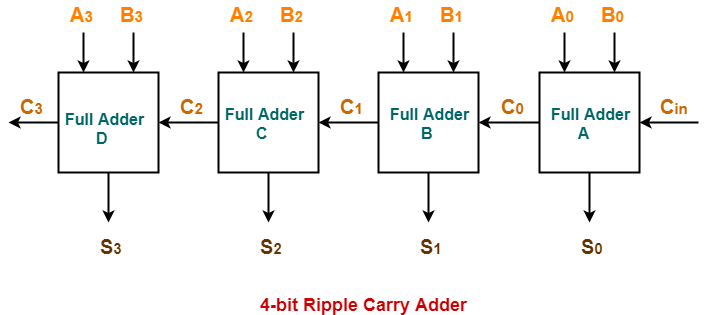• It generates the carry-in of each full adder simultaneously without causing any delay.
• The time complexity of carry look ahead adder = Θ (logn).

## Logic Diagram-

The logic diagram for carry look ahead adder is as shown below-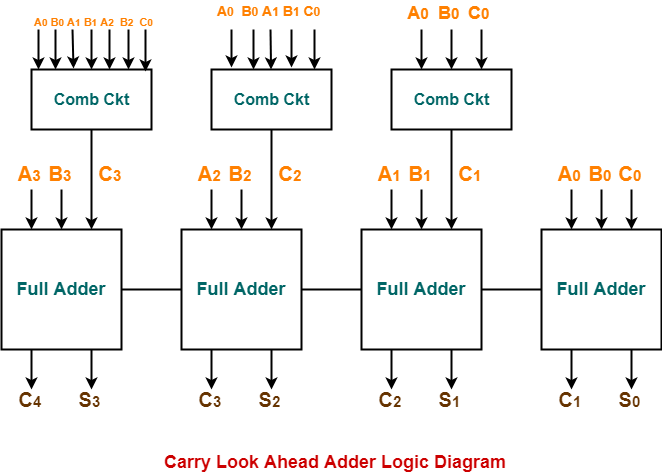The working of carry look ahead adder is based on the principle-The carry-in of any stage full adder is independent of the carry bits generated during intermediate stages.

The carry-in of any stage full adder depends only on the following two parameters-

• Bits being added in the previous stages
• Carry-in provided in the beginning

Now,

• The above two parameters are always known from the beginning.
• So, the carry-in of any stage full adder can be evaluated at any instant of time.
• Thus, any full adder need not wait until its carry-in is generated by its previous stage full adder.

Consider two 4-bit binary numbers A3A2A1A0 and B3B2B1B0 are to be added.

Mathematically, the two numbers will be added as-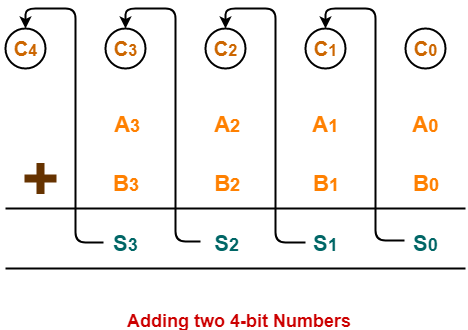From here, we have-

C1 = C0 (A0 ⊕ B0) + A0B0

C2 = C1 (A1 ⊕ B1) + A1B1

C3 = C2 (A2 ⊕ B2) + A2B2

C4 = C3 (A3 ⊕ B3) + A3B3

For simplicity, Let-

• Gi = AiBi where G is called carry generator
• Pi = Ai ⊕ Bi where P is called carry propagator

Then, re-writing the above equations, we have-

C1 = C0P0 + G………….. (1)

C2 = C1P1 + G1 ………….. (2)

C3 = C2P2 + G2 ………….. (3)

C4 = C3P3 + G3 ………….. (4)

Now,

• Clearly, C1, C2 and C3 are intermediate carry bits.
• So, let’s remove C1, C2 and C3 from RHS of every equation.
• Substituting (1) in (2), we get C2 in terms of C0.
• Then, substituting (2) in (3), we get C3 in terms of C0 and so on.

Finally, we have the following equations-

• C1 = C0P0 + G
• C2 = C0P0P1 + G0P1 + G1
• C3 = C0P0P1P2 + G0P1P2 + G1P2 + G2
• C4 =C0P0P1P2P3 + G0P1P2P3 + G1P2P3 + G2P3 + G3

These equations are important to remember.

These equations show that the carry-in of any stage full adder depends only on-

• Bits being added in the previous stages
• Carry bit which was provided in the beginning

### Trick To Memorize Above Equations-

As an example, let us consider the equation for generating carry bit C2.

There are three possible reasons for generation of C2 as depicted in the following picture-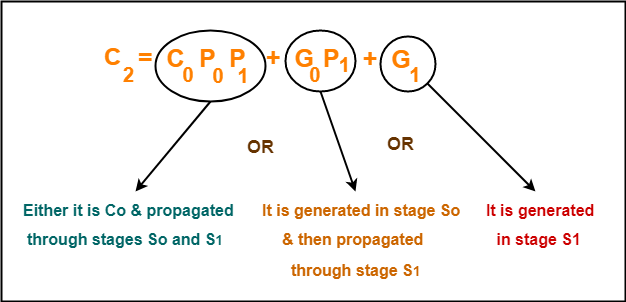In the similar manner, we can write other equations as well very easily.

## Implementation Of Carry Generator Circuits-

The above carry generator circuits are usually implemented as-

• Two level combinational circuits.
• Using AND and OR gates where gates are assumed to have any number of inputs.

### Implementation Of C1–

• The carry generator circuit for C1 is implemented as shown below.
• It requires 1 AND gate and 1 OR gate.

C1 = C0P0 + G0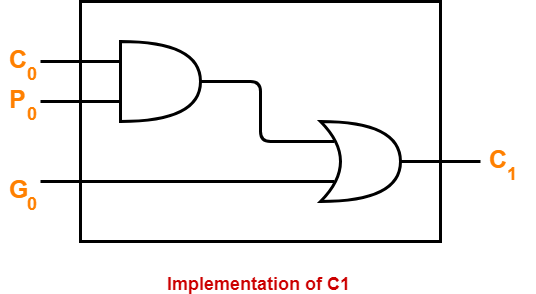### Implementation Of C2–

• The carry generator circuit for C2 is implemented as shown below.
• It requires 2 AND gates and 1 OR gate.

C2 = C0P0P1 + G0P1 + G1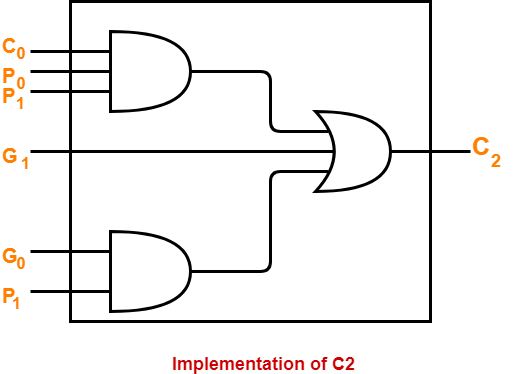### Implementation Of C3 & C4–

Similarly, we implement C3 and C4.

• Implementation of C3 uses 3 AND gates and 1 OR gate.
• Implementation of C4 uses 4 AND gates and 1 OR gate.

Total number of gates required to implement carry generators (provided carry propagators Pi and carry generators Gi) are-

• Total number of AND gates required for addition of 4-bit numbers = 1 + 2 + 3 + 4 = 10.
• Total number of OR gates required for addition of 4-bit numbers = 1 + 1 + 1 + 1 = 4.

### General Formula-

The following formula is used to calculate number of gates required for evaluating all carry bits-

 For a n-bit carry look ahead adder to evaluate all the carry bits, it requires-Number of AND gates = n(n+1) / 2Number of OR gates = n

• It generates the carry-in for each full adder simultaneously.
• It reduces the propagation delay.

• It involves complex hardware.
• It is costlier since it involves complex hardware.
• It gets more complicated as the number of bits increases.

Watch this Video Lecture

Next Article- K Maps | Karnaugh Maps

Get more notes and other study material of Digital Design.

Watch video lectures by visiting our YouTube channel LearnVidFun.

## Logic Gates-

Before you go through this article, make sure that you have gone through the previous article on Logic Gates.

We have discussed-

• Logic gates are the basic building blocks of any digital circuit.
• Logic gates are classified as-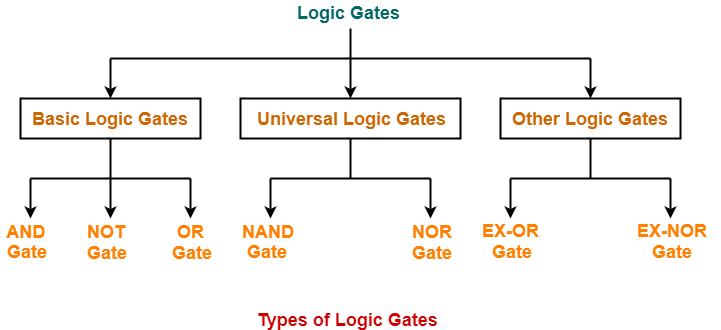## Alternative Logic Gates-

 Alternative logic gate is an alternate logic gate that produces the same output as the original logic gateand can be used during the unavailability of the original logic gate to serve the same purpose.

Alternative logic gates are also called as Alternate Gates.

Alternative logic gates are also called as Bubbled Gates since they contain bubbles in them.

The following table shows the original logic gate and its corresponding alternate gate-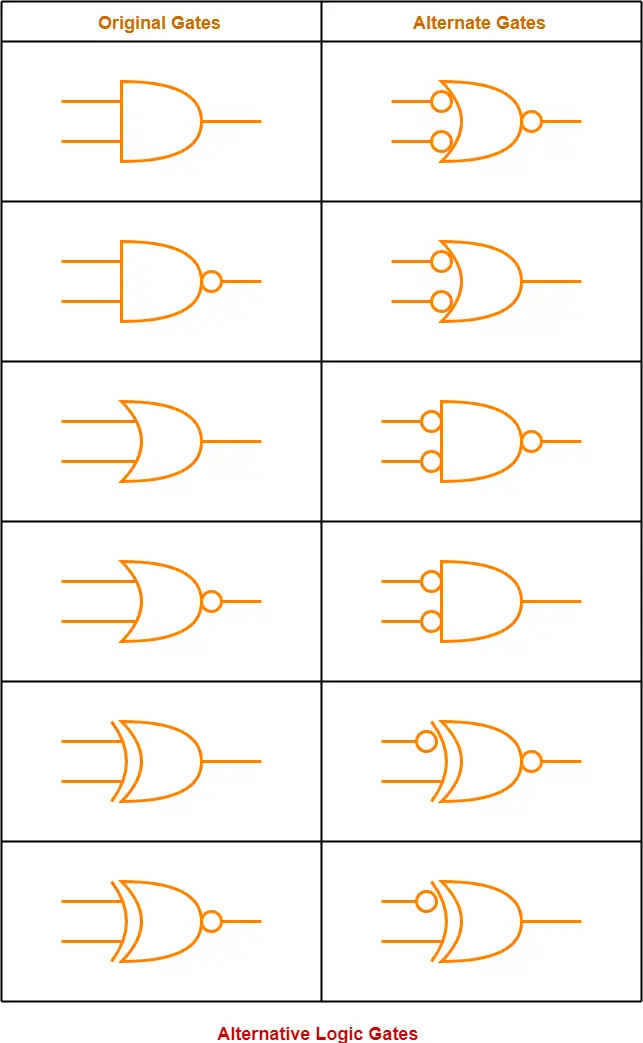## Rules To Memorize Alternative Logic Gates-

The following rules are helpful in drawing an alternate logic gate for a given logic gate-

#### For AND, NAND, OR & NOR Gates-

• Both the inputs of alternative gate will have bubbles (which represents NOT gate).
• For AND structured original gate, alternative gate will be OR structured.
• For OR structured original gate, alternative gate will be AND structured.
• If bubble is present at the output of original gate, then no bubble will be present at the output of alternative gate.
• If bubble is not present at the output of original gate, then a bubble will be present at the output of alternative gate.

#### For EX-OR & EX-NOR Gates-

• One of the inputs of alternative gate will have a bubble (which represents NOT gate).
• For EX-OR structured original gate, alternative gate will be EX-NOR structured.
• For EX-NOR structured original gate, alternative gate will be EX-OR structured.
• If bubble is present at the output of original gate, then no bubble will be present at the output of alternative gate.
• If bubble is not present at the output of original gate, then a bubble will be present at the output of alternative gate.

To gain better understanding about Alternative Logic Gates,

Watch this Video Lecture

Next Article- Latches & Flip-Flops

Get more notes and other study material of Digital Design.

Watch video lectures by visiting our YouTube channel LearnVidFun.

## Dual Of Boolean Expression-

To get a dual of any Boolean Expression, replace-

• OR with AND i.e. + with .
• AND with OR i.e. . with +
• 1 with 0
• 0 with 1

### Dual of Boolean Expression Examples-

Following are examples of dual of Boolean Expressions-

#### Example-01:

• Consensus  theorem is xy + x’z + yz = xy + x’z
• Dual of Consensus theorem is (x + y)(x’ + z)(y + z) = (x + y)(x’ + z)

#### Example-02:

• Boolean expression is xyz + x’yz’ + y’z = 1
• Dual of the above Boolean expression is (x + y + z)(x’ + y + z’)(y’ + z) = 0

## Self-Dual Functions-

 When a function is equal to its dual, it is called as a Self dual function.

### Example-

Consider the function : F (A , B , C) = AB + BC + CA

The dual of this function is-

Fd (A , B , C)

= (A + B)(B + C)(C + A)

= AB + BC + CA

Clearly, F (A , B , C) = Fd (A , B , C)

∴ F (A , B , C) is a self-dual function.

## Conditions For Self-Dual Function-

The necessary and sufficient conditions for any function to be a self-dual function are-

• The function must be a Neutral Function.
• The function must not contain any mutually exclusive terms.

### Mutually Exclusive Terms

Consider we have any term X consisting of some variables.

Then, a term obtained by complementing each variable of term X is called as its mutually exclusive term.

### Examples-

• (ABC , A’B’C’) are mutually exclusive terms.
• (AB’C , A’BC’) are mutually exclusive terms.

## Number of Self-Dual Functions-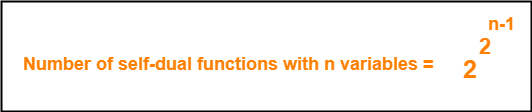Here n = number of Boolean variables in the function.

### Explanation-

• For a function to be a self-dual function, the function must be a neutral function.
• For a function to be a neutral function, number of minterms must be equal to number of maxterms.
• So, we choose half of the terms i.e. 2n / 2 = 2n-1 terms.
• Now, for each of these terms, we have two choices whether to include it or not in the self-dual function.

So, possible number of self-dual functions

= 2 x 2 x 2 x ……. x 2n-1

= 22^(n-1)

## Relationship Between Neutral Functions & Self-dual Functions-

• Every self-dual function is surely a neutral function.
• But every neutral function need not be a self-dual function.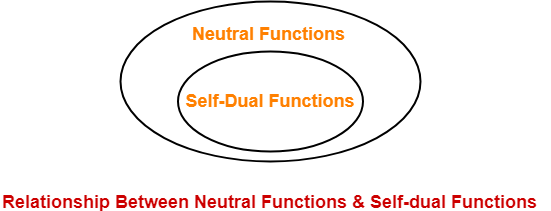## Important Property of Self-Dual Functions-

 Self-duality is closed under complementation.

### Example-

• If the function F (A , B , C) = ∑ (0 , 1 , 2 , 4) is a self-dual function.
• Then, its complement function F’ (A , B , C) = ∑ (3 , 5 , 6 , 7) will also be a self-dual function.

## Problem-

Consider the following functions-

1. F (A , B , C) = ∑ (0 , 2 , 3)
2. F (A , B , C) = ∑ (0 , 1 , 6 , 7)
3. F (A , B , C) = ∑ (0 , 1 , 2 , 4)
4. F (A , B , C) = ∑ (3 , 5 , 6 , 7)

Which of the above functions are self-dual functions?

1. Only (iii)
2. Only (ii)
3. Only (iii) and (iv)
4. All are self-dual functions

## Solution-

### Condition-01:

According to condition-01, for a function to be a self-dual function, the function must be a neutral function.

• In all the given options, we have functions of 3 variables- A, B and C.
• So, Neutral function must contain exactly 2n-1 = 23-1 = 4 minterms and 4 maxterms.
• But Function-(i) contains only 3 minterms. So, it is not a neutral function.
• Therefore, it can’t be a self-dual function and it gets eliminated.
• We are now left with three other functions which satisfies condition-01 and are all neutral functions.
• We will now use 2nd condition to eliminate the incorrect option(s).

### Condition-02:

According to condition-02, a self-dual function must not contain mutually exclusive terms.

First, let us find which terms are mutually exclusive-

 A B C Minterms 0 0 0 0 A’B’C’ 1 0 0 1 A’B’C 2 0 1 0 A’BC’ 3 0 1 1 A’BC 4 1 0 0 AB’C’ 5 1 0 1 AB’C 6 1 1 0 ABC’ 7 1 1 1 ABC

• From here, pairs of mutually exclusive terms are (0,7) , (1,6) , (2,5) , (3,4).
• Mutually exclusive terms are not allowed in self-dual functions.
• Therefore, terms inside the pairs can not appear together.
• But terms 0 and 7 appear together in the function-(ii).
• So, it can not be a self-dual function.
• But functions (iii) and (iv) do not contain any mutually exclusive terms.
• Therefore, functions (iii) and (iv) are self-dual functions.

Thus, Option (C) is correct.

### NOTE-

• Functions (iii) and (iv) are complementary functions.
• So, if one function is a self-dual function, the other function will also be a self-dual function.
• This is because self-dual functions are closed under complementation.

To gain better understanding about Self-Dual Functions,

Watch this Video Lecture

Get more notes and other study material of Digital Design.

Watch video lectures by visiting our YouTube channel LearnVidFun.

## Minterms and Maxterms-

 For any function consisting of n Boolean variables,Number of minterms possible = Number of maxterms possible = 2n

## Minterms and Maxterms Examples-

The examples of minterms and maxterms are-

### Example-01:

For any function consisting of 2 Boolean variables A and B, we have-

• Number of minterms possible = 22 =  4.
• Number of maxterms possible = 22 =  4.

The following table shows the minterms and maxterms-

 A B Minterms Maxterms 0 0 A’B’ A + B 0 1 A’B A + B’ 1 0 AB’ A’ + B 1 1 AB A’ + B’

### Example-02:

For any function consisting of 3 Boolean variables A, B and C, we have-

• Number of minterms possible = 23 =  8.
• Number of maxterms possible = 23 =  8.

The following table shows the minterms and maxterms-

 A B C Minterms Maxterms 0 0 0 A’B’C’ A + B + C 0 0 1 A’B’C A + B + C’ 0 1 0 A’BC’ A + B’ + C 0 1 1 A’BC A + B’ + C’ 1 0 0 AB’C’ A’ + B + C 1 0 1 AB’C A’ + B + C’ 1 1 0 ABC’ A’ + B’ + C 1 1 1 ABC A’ + B’ + C’

## Neutral Functions-

 A neutral function is a function for which number of minterms and number of maxterms are same.

### Example-

The following function is an example of a neutral function-

f( A, B) = A ⊕ B

This is because this function has equal number of minterms and maxterms as shown by the following table-

 A B A ⊕ B 0 0 0 0 1 1 1 0 1 1 1 0

## Number Of Neutral Functions Possible-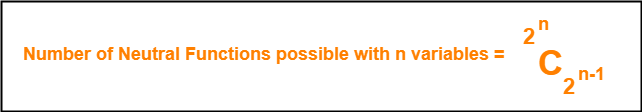Here n = Number of Boolean variables in the function.

### Explanation-

• For n variables, total number of terms possible = number of combinations of n variables = 2n.
• Since maximum number of terms possible = 2n, so we choose half of the terms i.e 2n / 2 = 2n-1.
• We assign them the output logic ‘1’.
• We assign ‘0’ to rest half of the terms.
• Thus, number of neutral functions possible with n Boolean variables = C ( 2n , 2n-1 ).

## Problem-

Consider any function consisting of 2 Boolean variables A and B-

1. Calculate the total number of neutral functions that are possible.
2. Write all the neutral functions.

### Solution-

We know, number of neutral functions possible with n Boolean variables = C ( 2n , 2n-1 ).

Substituting n = 2, we get-

Number of neutral functions possible with 2 Boolean variables

= C ( 22 , 22-1 )

= C ( 4 , 2 )

= 6

Thus, total 6 Boolean functions are possible with 2 Boolean variables.

The 6 Boolean Functions are-

• f ( A , B ) = A
• f ( A , B ) = A’
• f ( A , B ) = B
• f ( A , B ) = B’
• f ( A , B ) = A ⊙ B
• f ( A , B ) = A ⊕ B

 Variables Boolean Functions A B A A’ B B’ A⊙B A⊕B 0 0 0 1 0 1 1 0 0 1 0 1 1 0 0 1 1 0 1 0 0 1 0 1 1 1 1 0 1 0 1 0

The above table clearly shows that for each function, number of minterms = number of maxterms.

So, they all are neutral functions.

To gain better understanding about Neutral Functions,

Watch this Video Lecture

Next Article- Self-Dual Functions

Get more notes and other study material of Digital Design.

Watch video lectures by visiting our YouTube channel LearnVidFun.